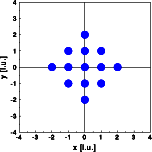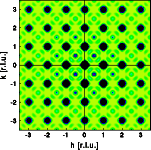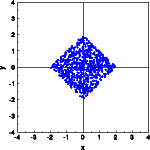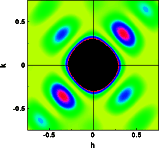Interactive Tutorial about Diffraction Convolution V: Box at 45 degreesConvolution Convolution theorem Square box Rectangular box Circular box Box at 45 degrees Goto Contents In the last example lets limit the crystal by a square box rotated around 45 degrees (left image). Again the reciprocal lattice results convoluted by the Fourier transform of a square box (right image). Click on the pictures to get a full screen version.As expected, the Bragg reflections are convoluted by the Fourier transform of the shape function again. This time the subsidiary maxima are positioned long the diagonal axes. These features represent the Fourier transform of the shape function. The box is simulated by filling the shape at random with a large number (800) of atoms (left image). The resulting electron density distribution fills the box fairly equally. Next the Fourier transform is calculated in a small section of reciprocal space near the origin. The calculated intensity distribution corresponds to the distribution of the subsidiary maxima observed in the Fourier transform above.The deviations from square symmetry are due to the limited statistics provided by the 800 atoms within the square box. © Th. Proffen and R.B. Neder, 2003